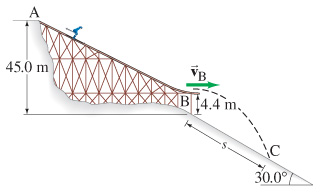Physics Practice Problems Solving Projectile Motion Using Energy Practice Problems Solution: A 63 kg skier starts from rest at the top of a ski...

🤓 Based on our data, we think this question is relevant for Professor Potvin's class at FIU.

# Solution: A 63 kg skier starts from rest at the top of a ski jump, point A in the figure, and travels down the ramp. If friction and air resistance can be neglected, determine(a) her speed vB when she reaches the horizontal end of the ramp at B(b) the distance s to where she strikes the ground at C

###### Problem

A 63 kg skier starts from rest at the top of a ski jump, point A in the figure, and travels down the ramp. If friction and air resistance can be neglected, determine
(a) her speed vB when she reaches the horizontal end of the ramp at B
(b) the distance s to where she strikes the ground at CSolving Projectile Motion Using Energy

Solving Projectile Motion Using Energy

#### Q. A film of Jesse Owenss famous long jump in the 1936 Olympics shows that his center of mass rose 1.1 m from launch point to the top of the arc. What mi...

Solved • Wed Oct 31 2018 14:27:58 GMT-0400 (EDT)

Solving Projectile Motion Using Energy

#### Q. A projectile is fired at an upward angle of 48.0° from the top of a 130-m-high cliff with a speed of 180 m/s m m/s. What will be its speed when it str...

Solved • Wed Oct 31 2018 14:27:56 GMT-0400 (EDT)

Solving Projectile Motion Using Energy

#### Q. A 62-kg skier starts from rest at the top of a ski jump, point A in the figure , and travels down the ramp.If friction and air resistance can be negle...

Solved • Wed Oct 31 2018 14:27:56 GMT-0400 (EDT)

Solving Projectile Motion Using Energy

#### Q. In the high jump, the kinetic energy of an athlete is transformed into gravitational potential energy without the aid of a pole.With what minimum spee...

Solved • Wed Oct 31 2018 14:27:50 GMT-0400 (EDT)